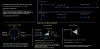# GMLCalculating a non-axis aligned bounce/reflection vector for collision resolution

#### StubbinMyToe

##### Member
Non-axis aligned 2D reflection vector

GM Version:
2.3 (demo is for 2.3 but the concept would work in any version)
Target Platform: All
Download: A link to the freely available source code and project is available here:
Links: Tutorial Video and Github link

Summary

This video tutorial builds upon our vector knowledge of the dot product by explaining how it can be used to calculate a collision reflection vector we can use to bounce off surfaces that are not axis aligned.

Over the past few days I created a series of 2D vector tutorial videos here: 2D Vector Basics - Tutorial Playlist

In that playlist I discuss the dot product and how it can be used. Here is a screenshot from the dot product video, notice the bottom right discusses the reflection formula:To make that reflection formula easy to visualize, I created a very simple GameMaker 2.3 GML based demo to show how it works.
This tutorial is here:

Source Code

The full source and project is available at the tutorial link. For those who are interested in the reflection formula, here is the main GML code that will perform it. Although my demo is contained within a struct, the algorithm boils down to these two methods:

GML:
``````// Dot product of two vectors
// If the dot product is 0, the vectors are perpendicular/orthogonal
// If the dot product is positve, the vectors are pointing in similar directions(acute angles).
// If the dot product is negative, the vectors are pointing in nearly opposite directions(obtuse angles).
//
// Note: Any vector dotted with itself (AdotA) gives us its magnitude squared.
// So if A is a unit vector, AdotA = 1
/// @func function dotV2D(_v1, _v2)
/// @desc Return the dot product of two vectors _v1 dot _v2
/// @param {V2D} _v1 First vector
/// @param {V2D} _v2 Second vector
/// @return {real} The dot product (0 = perpendicular,postive if within 180 degrees, negative if > 180 degrees
function dotV2D(_v1, _v2)
{
return _v1.x * _v2.x + _v1.y * _v2.y;
}

/// Reflect vector _V off surface vector _N (useful for a bounce vector)
/// @func reflectV2D(_V, _N, _N_Is_UnitVector)
/// @desc Reflect vector _V off surface vector _N
/// @param {V2D} _V Vector you want to reflect onto _N
/// @param {V2D} _N Vector you want to reflect onto (usually this is a surface normal)
/// @return {bool} _N_Is_UnitVector True if _N is a unit vector (faster if it is)
/// @return {V2D} The resulting reflection vector
function reflectV2D(_V, _N, _N_Is_UnitVector)
{
// Formula = V - 2(VdotN / NdotN)N
var scalar;

if ( !_N_Is_UnitVector )
scalar = 2 * (dotV2D(_V, _N) / dotV2D(_N, _N));
// If _N is a unit vector, we can simplify the math!
else
scalar = 2 * dotV2D(_V, _N);

return new V2D( _V.x - (_N.x * scalar), _V.y - (_N.y * scalar));
}``````
Conclusion

I hope this helps new game programmers learn how to make their collisions respond more realistically, and if you have any suggestions I would love to hear them!

#### samspade

##### Member
This is great. Vectors are one of the first things I learned when I started coding. I return to them periodically, and I always have to relearn projection, reflection, and rotate.

The ability to use structs make them much easier. Any reason you didn't make the stuct methods static here?

•StubbinMyToe

#### StubbinMyToe

##### Member
This is great. Vectors are one of the first things I learned when I started coding. I return to them periodically, and I always have to relearn projection, reflection, and rotate.

The ability to use structs make them much easier. Any reason you didn't make the stuct methods static here?
Thanks! They should be static method variables, you're right.

#### Bentley

##### Member
Thanks for the tutorial!